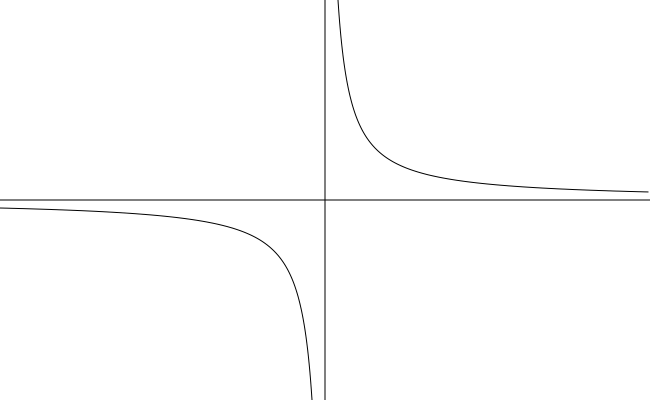# Section 3.5 - Rational Functions and Asymptotes

Section Objectives

1. Determine the vertical, horizontal, and/or slant asymptotes of the graph of a rational function.
2. Sketch the graph of a rational function.

### Rational Functions

A rational function is a ratio of two polynomials. That is, a rational function $R$ is a function of the form

$\displaystyle R(x)=\frac{P(x)}{Q(x)},$

where $P$ and $Q$ are polynomials and $Q(x) \ne 0$.

The domain of a rational function is the set of all real numbers for which the denominator is nonzero.

Here are some examples of rational functions...

• $\displaystyle g(x)=\frac{x^3-x^2+x-1}{x^2+1}$
• $\displaystyle y=\frac{5x-7}{x}$
• $y=x^5+2$
• $f(x)=5$

### Reciprocal function

One of the simplest rational functions is the reciprocal function $\displaystyle y = \frac{1}{x}$.• Domain $=(-\infty,0) \cup (0,\infty)$
• Range $=(-\infty,0) \cup (0,\infty)$
• The reciprocal function is an odd function. Its graph is symmetric about the origin.
• As $x \to \pm \infty$, $\quad y \to 0$. (The $x$-axis is a horizontal asymptote of the graph.)
• As $x \to 0^{+/-}$, $\quad y \to \pm \infty$. (The $y$-axis is a vertical asymptote of the graph.)

### Asymptotes

The vertical line $x=a$ is a vertical asymptote of the graph of $f$ if the $y$-values of $f(x)$ grow without bound (positively or negatively) as the $x$-values get closer and closer to $a$ (from either side).

IMPORTANT IDEA: The zeros of the denominator of a rational function give possible vertical asymptotes...

• The rational function $f(x)=p(x)/q(x)$ has a vertical asymptote at $x=a$ if $q(a) = 0$ and $p(a) \ne 0$.
• If $q(a)=0$ and $p(a)=0$, simplify the rational expression, and then check again for the zeros of the denominator.

#### Examples

• Let $\displaystyle f(x)= \frac{x^2+1}{(x-3)(x+2)}$. Determine the vertical asymptotes of the graph of $f$.

• Let $\displaystyle g(x)= \frac{2x-2}{(x-1)(x-5)}$. Determine the vertical asymptotes of the graph of $g$.

The line $y=b$ is a horizontal asymptote of the graph of $f$ if the $y$-values of $f(x)$ get closer and closer to the number $b$ as the $x$-values grow without bound (positively or negatively).

IMPORTANT IDEA: Horizontal asymptotes can be found by examining the degrees of the numerator and denominator polynomials.

• If the degrees are equal, the horizontal asymptote is $y=a/b$, where $a$ and $b$ are the leading coefficients of the numerator and denominator, respectively.
• If the degree of the denominator is greater than the degree of the numerator, $y=0$ is the horizontal asymptote.
• If the degree of the numerator is greater than the degree of the denominator, the graph has no horizontal asymptote.

While the graph of a rational function can have any number of vertical asymptotes, it can have at most one horizontal asymptote. (In general, functions can have at most two horizontal asymptotes---but rational functions can have at most one!)

#### Examples

• Determine the horizontal asymptote of the graph of $\displaystyle R(x)=\frac{3x^2+2x-9}{2x^2-x-1}$.

• Determine the horizontal asymptote of the graph of $\displaystyle H(x)=\frac{3x}{6x^2+3x+112}$.

• Explain how you know that the graph of $\displaystyle f(x)=\frac{x^3-8}{x+7}$ has no horizontal asymptote.

A non-horizontal or non-vertical line that a graph approaches as the $x$-values grow without bound is called a slant (or oblique) asymptote.

• The graph of a rational function has a slant asymptote if the degree of the numerator is exactly one more than the degree of the denominator.
• To determine an equation for the slant asymptote, carry out long division: after long division, if the quotient is $y=ax+b$, then $y=ax+b$ is an equation of the slant asymptote.

#### Example

• Let $\displaystyle f(x)= \frac{x^3+3x^2+1}{x^2-x}$. Determine the slant asymptote and the vertical asymptotes of the graph of $f$.

### Graphing Rational Functions

To graph the rational function $f(x)$:

1. Completely factor the denominator and specify all real numbers excluded from the domain of $f$ (restricted values).
2. Completely factor the numerator and cancel any factors common to both the numerator and the denominator. (If any factors cancel, your graph will have holes at the corresponding $x$-values.)
3. Examine the factors that remain in the denominator. Then find and sketch the vertical asymptotes. (Sketch with a dashed line.)
4. Find and sketch (dashed) any horizontal or slant asymptotes.
5. Determine the $x$- and $y$-intercepts of the graph.
6. To determine when the graph is above or below the $x$ axis, construct a sign chart: Away from your graph, sketch a number line separated (in order) by the $x$-values associated with the vertical asymptotes, $x$-intercepts, and other points excluded from the domain. Mark which is which. Then determine and mark the signs ($+$ or $-$) of $f$ on each interval of your number line.
7. Determine $x$-values (if any) where the graph crosses the horizontal or slant asymptotes.
8. Plot some points.
9. Determine if there is symmetry in the graph.
10. Use all the information above to sketch the graph. Use your graphing calculator as an aid.

#### Examples

• Sketch the graph of $\displaystyle y=\frac{2x-4}{x-5}$.

• Sketch the graph of $\displaystyle y=\frac{x^2-2x-3}{2x+6}$.